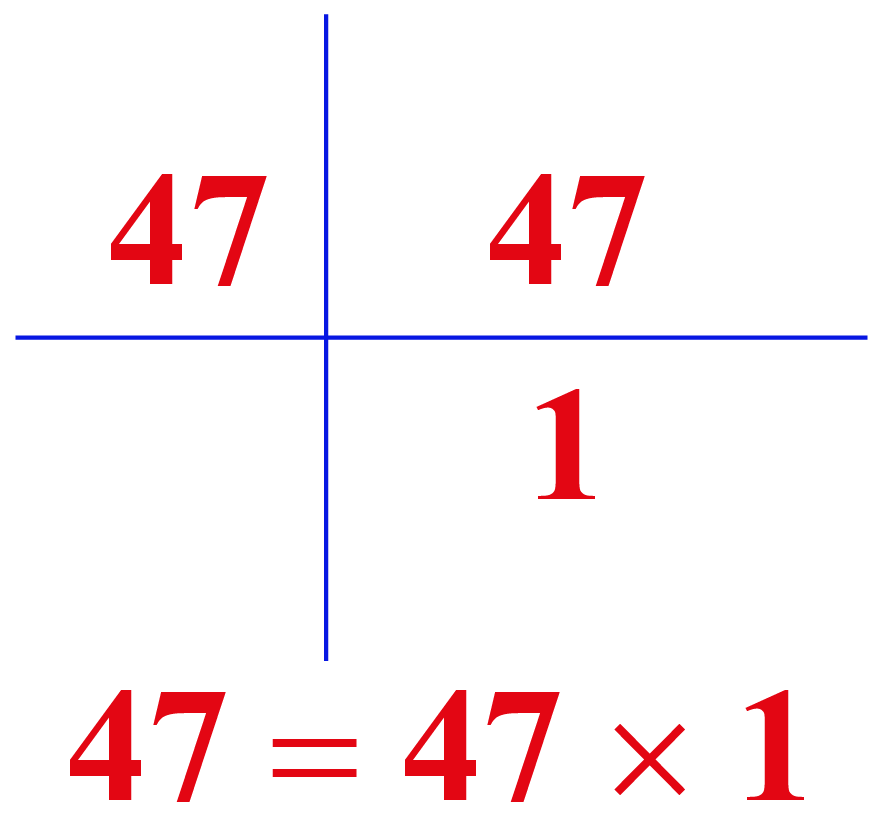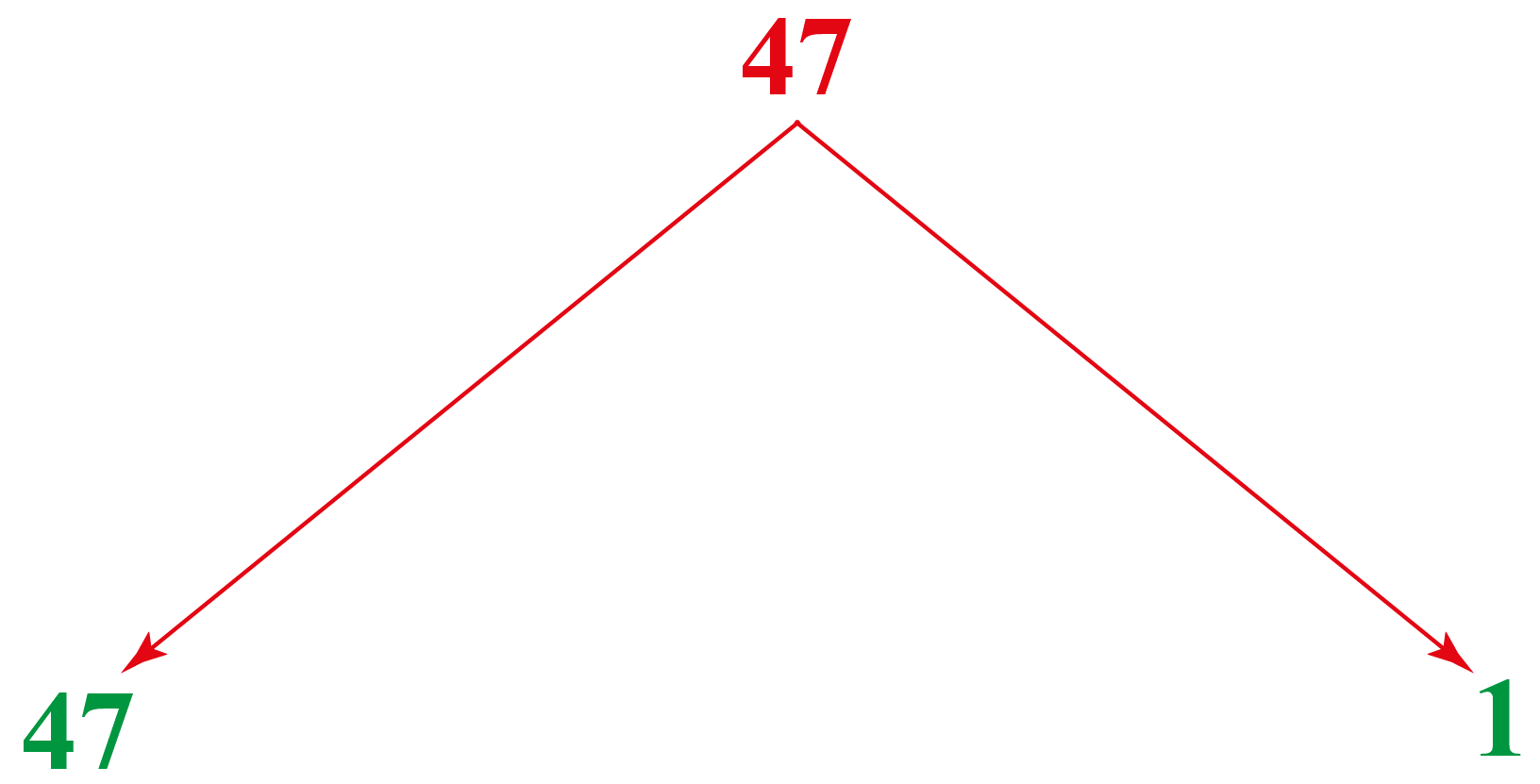# Is 47 A Prime Number?

Is 47 A Prime Number?
Go back to  'Prime Numbers'

Do you know that this famous Hot Spring National Park in the United States of America has a total of 47 hot springs?Another fact that we're going to study today is that 47 is a prime number since it has only two factors: 1 and the number itself.This chapter will explore other topics like prime numbers, is 97 a prime number, is 49 a prime number, is 57 a prime number, and is 91 a prime number in the concept of is 47 a prime number.

Check out the interactive questions to know more about the lesson and try your hand at solving a few interesting practice questions at the end of the page.

## What Is a Prime Number?

Any whole number which is greater than 1, and has only 2 factors, 1 and the number itself, is called a prime number.The number clearly has only two factors, 1 and the number 47

Thus, observing the factors of 47, we conclude that it is a prime number.

Examples:

Let us see a few more examples.

• Factors of number 97 = 1, 97. So, is 97 a prime number? Yes, because it has only 2 factors.
• Factors of number 57 = 1, 57. So, is 57 a prime number? Yes, because it has only 2 factors.
• Factors of number 91 = 1, 7, 13, 91. So, is 91 a prime number? No, because it has 4 factors.
• Factors of number 49 = 1, 7, 49. So, is 49 a prime number? No, because it has 3 factors.

## Is 47 a Prime Number?

To check if a number is prime or not, we find out all the factors of the number.

This can be done using different methods:

• Divison of the number by all the prime numbers starting from 1
• By prime factorization

After following one of the above steps, we find all the possible factors of the number and thus conclude if the number is prime or not.

Let us check this for 47:

Observe the figure which shows the prime factorization of 47:The prime factors of 47 = 1 and 47

Thus, even by repeated multiplication of its prime factors, 47 has only two factors in all = 1 and 47

Hence, 47 is a prime number.

## Solved Examples

 Example 1

Loren is preparing a list of all prime numbers. Can you help her find the number of prime numbers that are less than 47?

Solution

All the prime numbers less than 47 = 2, 3, 5, 7, 11, 13, 17, 19, 23, 29, 31, 37, 41, 43

The number of prime numbers less than 47 is 14

 $$\therefore$$ The number of prime numbers less than 47 are 14
 Example 2

Joel needs to prove that 1 added to or subtracted from 47 makes it a composite number. How will he prove it?

Solution

On adding 1 to 47, Joel gets 48

Factors of 48 = 1, 2, 3, 4, 6, 8, 12, 16, 24, and 48

Clearly, 48 has more than 2 factors. Thus, it is not a prime number.

On subtracting 1 from 47, he gets 46

Factors of number 46 = 1, 2, 23, and 46

It has factors other than 1 and 46, which makes it a composite number.

Hence, 1 added to or subtracted from 47 makes it a composite number.

 $$\therefore$$1 added to or subtracted from 47 makes it a composite number.

## Interactive Questions

Here are a few activities for you to practice.Challenging Questions
• Leslie needs to find those prime numbers between 1 to 100 whose sum also gives a prime number. Can you help him?
• How will Jamie check if 51 is a prime number or not?

## Let's Summarize

The mini-lesson targeted the fascinating concept of the "Is 47 a prime number". The math journey around the "Is 47 a prime number" starts with what a student already knows, and goes on to creatively crafting a fresh concept in the young minds. Done in a way that not only it is relatable and easy to grasp, but also will stay with them forever. Here lies the magic with Cuemath.

At Cuemath, our team of math experts is dedicated to making learning fun for our favorite readers, the students!

Through an interactive and engaging learning-teaching-learning approach, the teachers explore all angles of a topic.

Be it worksheets, online classes, doubt sessions, or any other form of relation, it’s the logical thinking and smart learning approach that we, at Cuemath, believe in.

## 1. What can 47 be divided by?

47 is a prime number, which means it has only two factors 1 and 47. Thus, it can only be divided by 1 and 47

## 2. Is 47 a prime number? Yes or no?

Yes, 47 is a prime number because it has only two factors, 1 and the number itself.

Factors of 47 = 1 and 47

## 3. Is 47 a perfect square?

The square root of 47 = $$\sqrt{47}$$ = 6.85565, which is not a whole number.

Thus, 47 is not a perfect square.

More Important Topics
Numbers
Algebra
Geometry
Measurement
Money
Data
Trigonometry
Calculus